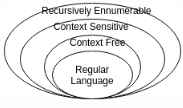Courses

# Test: Context Free Grammar Derivations & Definitions

## 10 Questions MCQ Test Theory of Computation | Test: Context Free Grammar Derivations & Definitions

Description
This mock test of Test: Context Free Grammar Derivations & Definitions for Computer Science Engineering (CSE) helps you for every Computer Science Engineering (CSE) entrance exam. This contains 10 Multiple Choice Questions for Computer Science Engineering (CSE) Test: Context Free Grammar Derivations & Definitions (mcq) to study with solutions a complete question bank. The solved questions answers in this Test: Context Free Grammar Derivations & Definitions quiz give you a good mix of easy questions and tough questions. Computer Science Engineering (CSE) students definitely take this Test: Context Free Grammar Derivations & Definitions exercise for a better result in the exam. You can find other Test: Context Free Grammar Derivations & Definitions extra questions, long questions & short questions for Computer Science Engineering (CSE) on EduRev as well by searching above.
QUESTION: 1

### The entity which generate Language is termed as:

Solution:

The entity which accepts a language is termed as Automata while the one which generates it is called Grammar. Tokens are the smallest individual unit of a program.

QUESTION: 2

### Production Rule: aAb->agb belongs to which of the following category?

Solution:

Context Sensitive Language or Type 1 or Linearly Bounded Non deterministic Language has the production rule where the production is context dependent i.e. aAb->agb.

QUESTION: 3

### Which of the following statement is false?

Solution:

Explanation: Every regular language can be produced by context free grammar and context free language can be produced by context sensitive grammar and so on.QUESTION: 4

The Grammar can be defined as: G=(V, ∑, p, S)In the given definition, what does S represents?

Solution:

G=(V, ∑, p, S), here V=Finite set of variables, ∑= set of terminals, p= finite productions, S= Starting Variable.

QUESTION: 5

Which among the following cannot be accepted by a regular grammar?

Solution:

There exists no finite automata to accept the given language i.e. 0n1n. For other options, it is possible to make a dfa or nfa representing the language set.

QUESTION: 6

Which of the expression is appropriate?For production p: a->b where a∈V and b∈_______

Solution:

According to the definition, the starting variable can produce another variable or any terminal or a variable which leads to terminal.

QUESTION: 7

For S->0S1|e for ∑={0,1}*, which of the following is wrong for the language produced?

Solution:

L={e, 01, 0011, 000111, ……0n1n }. As epsilon is a part of the set, thus all the options are correct implying none of them to be wrong.

QUESTION: 8

The minimum number of productions required to produce a language consisting of palindrome strings over ∑={a,b} is

Solution:

The grammar which produces a palindrome set can be written as:
S-> aSa | bSb | e | a | b
L={e, a, b, aba, abbbaabbba…..}

QUESTION: 9

Which of the following statement is correct?

Solution:

Regular grammar is a subset of context free grammar and thus all regular grammars are context free.

QUESTION: 10

Are ambiguous grammar context free?

Solution:

A context free grammar G is ambiguous if there is atleast one string in L(G) which has two or more distinct leftmost derivations.